2018-12-28 11:37:04 qq_33414271 阅读数 264
• ###### 基于深度学习实现场景判断模型和算法优化

本次课程诚邀PowerAI人工智能马拉松编程大赛获奖前三名团队精英，在线分享大赛获奖作品算法/模型等技术干货。

3682 人正在学习 去看看 CSDN讲师

# 一、发展背景及基本框架

## 最基本的框架——梯度下降法（GD）

(1) 计算目标函数关于参数的梯度
$g_t = \nabla_\theta J(\theta)$
(2) 根据历史梯度计算一阶和二阶动量
$m_t = \phi(g_1, g_2, \cdots, g_t)$
$v_t = \psi(g_1, g_2, \cdots, g_t)$
(3) 更新模型参数
$\theta_{t+1} = \theta_t - \frac{1}{\sqrt{v_t + \epsilon}} m_t$

# 二、GD算法体系“长谷 （Long Valley）”点——没有基于梯度信息缩放的算法在这里很难打破对称性——SGD在哪里都找不到， Nesterov Accelerated Gradient（NAG） / Momentum 在优化方向上建立速度之前会出现振荡。（此时自适应算法会更好）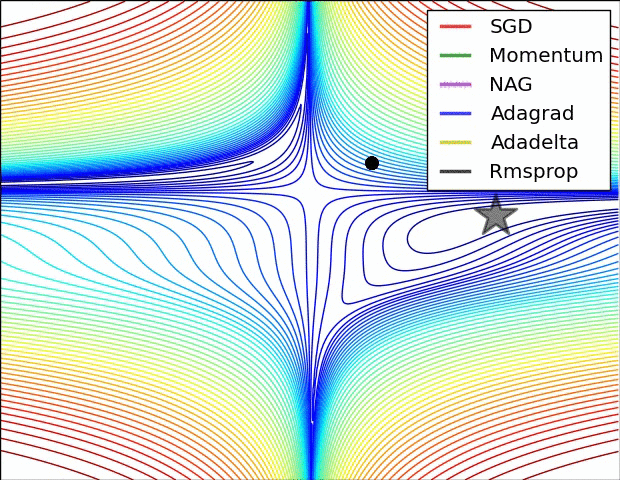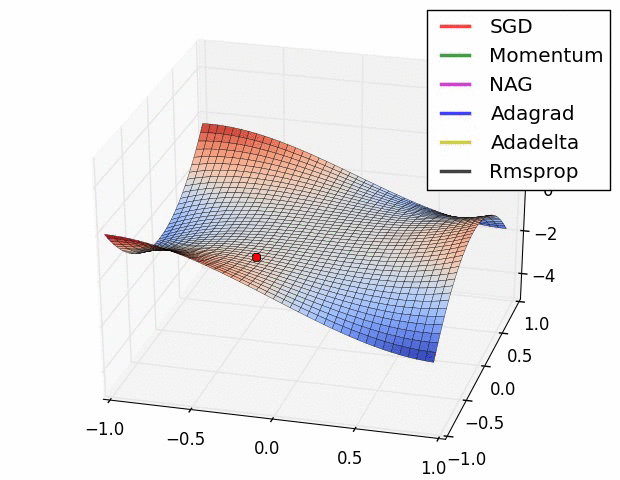NAG /Momentum探索周围，几乎走了不同的路径。

# 2.1-朴素 SGD (Stochastic Gradient Descent)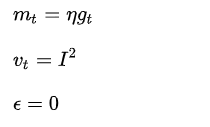SGD 的缺点在于收敛速度慢，可能在长谷处震荡。并且，如何合理的选择学习率是 SGD 的一大难点。

# 2.2-SGD with Momentum

$m_t = \gamma m_{t-1} + \eta g_t$

SGD-M 在原步长之上，增加了与上一时刻步长相关的 $\gamma m_{t-1}$$\gamma$ 通常取 0.9 左右。这意味着参数更新方向不仅由当前的梯度决定，也与此前累积的下降方向有关。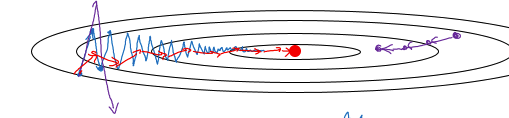NAG 即是为此而设计的，其在 SGD-M 的基础上进一步改进了步骤 1 中的梯度计算公式：
$g_t = \nabla_\theta J(\theta - \gamma m_{t-1})$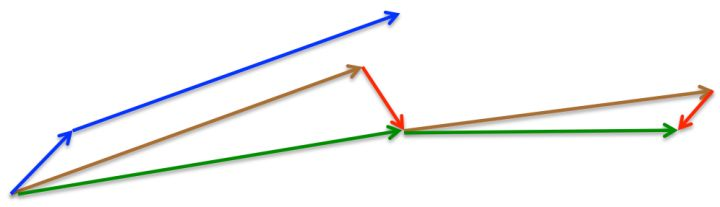SGD-M 的步长计算了当前梯度（短蓝向量）和动量项 （长蓝向量）。然而，既然已经利用了动量项来更新 ，那不妨先计算出下一时刻 $\theta$ 的近似位置 （棕向量），并根据该未来位置计算梯度（红向量），然后使用和 SGD-M 中相同的方式计算步长（绿向量）。这种计算梯度的方式可以使算法更好的「预测未来」，提前调整更新速率。

SGD、SGD-M 和 NAG 均是以相同的学习率去更新 $\theta$ 的各个分量。而深度学习模型中往往涉及大量的参数，不同参数的更新频率往往有所区别。

$v_t = \text{diag}(\sum_{i=1}^t g_{i,1}^2, \sum_{i=1}^t g_{i,2}^2, \cdots, \sum_{i=1}^t g_{i,d}^2)$

# 2.5-RMSprop

$g_t \odot g_t$$g_t^2$ ，有
$v_t = \gamma v_{t-1} + (1-\gamma) \cdot \text{diag}(g_t^2)$

CSC321 Neural Networks for Machine Learning - Lecture 6a

Adam: A Method for Stochastic Optimization

$m_t = \eta[ \beta_1 m_{t-1} + (1 - \beta_1)g_t ]$
$v_t = \beta_2 v_{t-1} + (1-\beta_2) \cdot \text{diag}(g_t^2)$

$m_0 = 0$
$v_0 = 0$

$\hat{m}_t = \frac{m_t}{1-\beta_1^t}$
$\hat{v}_t = \frac{v_t}{1-\beta_2^t}$

$\theta_{t+1} = \theta_t - \frac{1}{\sqrt{\hat{v}_t} + \epsilon } \hat{m}_t$

# 三、小结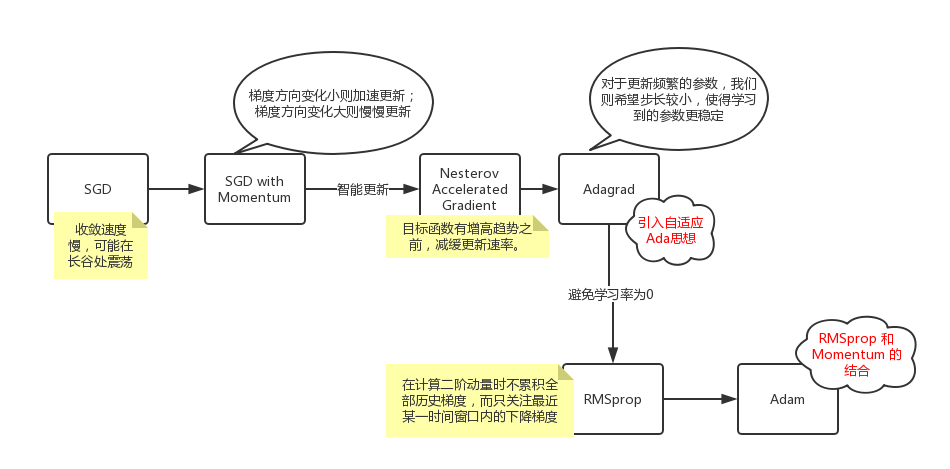从 SGD 到 Adam —— 深度学习优化算法概览(一) - 骆梁宸的文章 - 知乎
https://zhuanlan.zhihu.com/p/32626442
https://zhuanlan.zhihu.com/p/32230623
https://blog.csdn.net/u012223913/article/details/78432412
Visualizing Optimization Algos

2019-05-17 12:07:02 weixin_37142859 阅读数 103
• ###### 基于深度学习实现场景判断模型和算法优化

本次课程诚邀PowerAI人工智能马拉松编程大赛获奖前三名团队精英，在线分享大赛获奖作品算法/模型等技术干货。

3682 人正在学习 去看看 CSDN讲师

# 深度学习优化算法

## 1梯度下降算法的三个变种

$\theta=\theta-\eta \cdot \nabla_{\theta} J(\theta)$

(打公式我有一个独特的窍门，嘻嘻)

• 能保证模型最后收敛于全局最小点（若损失为凸）或局部最小点（损失函数非凸)

• 每更新一次参数需要计算出整个数据集每一个样本的推断结果并计算损失，计算量大，内存占用大
• 权重更新缓慢
• 无法在线学习

$\theta=\theta-\eta \cdot \nabla_{\theta} J\left(\theta ; x^{(i)} ; y^{(i)}\right)$

• 研究表明，当学习率较小时，SGD和BGD有相同的收敛点
• 在线学习

• SGD由于其频繁的权重更新，会导致损失在下降过程中出现较大的波动。但是波动可能使损失函数跳出局部最小值，进入一个小的收敛点。

$\theta=\theta-\eta \cdot \nabla_{\theta} J\left(\theta ; x^{(i : i+n)} ; y^{(i : i+n)}\right)$

• 减小了权重更新的方差，使得损失收敛于一个更稳定的点
• mini-batch的使用使得我们最大化利用GPU的并行计算能力

## 2 梯度下降算法的痛点

• 学习率大小选择困难。太小导致训练缓慢，太大则容易导致难以收敛，在（局部）最小点附近波动
• 算法中所有的参数都使用相同的学习率进行更新，但实际上对于不同的参数，可能有不同的更新需求
• 在高维的损失优化中，很难收敛到每一维数据梯度都接近于0的点，相反，很容易收敛于鞍点或局部最小点

## 3 各种梯度下降算法

### Momentum

\begin{aligned} v_{t} &=\gamma v_{t-1}+\eta \nabla_{\theta} J(\theta) \\ \theta &=\theta-v_{t} \end{aligned}

\begin{aligned}v_t &= \gamma v_{t-1} + \eta g_t \\&=\eta g_t + \gamma(\eta g_{t-1}+\gamma v_{t-2})\\&=\eta g_t + \gamma \eta g_{t-1} + \gamma^2v_{t-2}\\ &...\\&=\eta g_t+\gamma \eta g_{t-1}+\gamma ^2 \eta g_{t-2} + ...+\gamma ^ {t-1}\eta g_1\end{aligned}

• 若在某个维度多次权重更新方向一致，则会加速该权重的更新
• 若在某个维度权重更新方向一直发生变化，即梯度出现正负交替，多个梯度求和的结果则会减小该方向权重的变化。

$\begin{array}{l}{v_{t}=\gamma v_{t-1}+\eta \nabla_{\theta} J\left(\theta-\gamma v_{t-1}\right)} \\ {\theta=\theta-v_{t}}\end{array}$

• 累计动量项:$\gamma v_{t-1}$
• 损失梯度项:
$\eta \nabla_{\theta} J\left(\theta-\gamma v_{t-1}\right)$(Nesterov);
$\eta \nabla_{\theta} J(\theta)$(Momentum)

• Momentum:计算当前位置损失的梯度（仍与之前的梯度相同），结合累计动量项，更新权重，最终导致损失反而变大
• Nesterov:计算下一位置的近似梯度(过了最小点之后，此时的梯度与之前相反)，结合累计动量项，更新权重。由于损失梯度项变为相反值，一定程度上减少了权重更新的幅度，缓和甚至避免了损失的回升！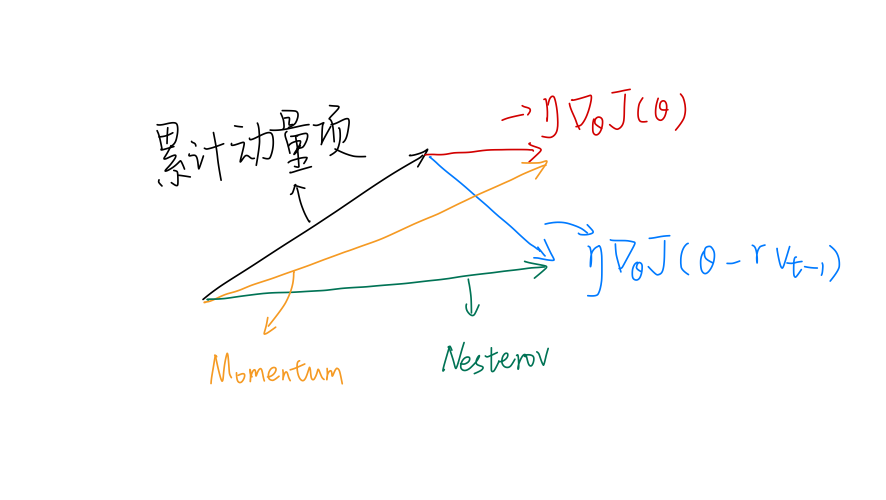Momentum的损失梯度项（红色）垂直方向的分量接近0，但累计动量项仍有很大的垂直分量，两个分量合成后（黄色），垂直方向的权重仍进行了很大的权重更新。
Nesterov的损失梯度项是下一位置的近似梯度，由于下一位置梯度和当前相反，因此垂直分量向下。两个分量合成后，垂直方向的分量抵消了一部分，最终垂直方向的权重更新不大。

$\theta_{t+1, i}=\theta_{t, i}-\frac{\eta}{\sqrt{G_{t, i i}+\epsilon}} \cdot g_{t, i}$

• $\theta_{t+1,i}$$t+1$时刻$i$方向的权重；
• $G_{t,ii}$是对角阵$G_t$对角上第$i$个元素，该元素大小为$\theta_i$以往每一次梯度的平方和;
• $\epsilon=10^{-8}$,避免除0

$E\left[g^{2}\right]_{t}=\gamma E\left[g^{2}\right]_{t-1}+(1-\gamma) g_{t}^{2}$

$\Delta \theta_{t}=-\frac{\eta}{\sqrt{E\left[g^{2}\right]_{t}+\epsilon}} g_{t}$

$\Delta \theta_{t}=-\frac{\eta}{R M S[g]_{t}} g_{t}$

$E\left[\Delta \theta^{2}\right]_{t}=\gamma E\left[\Delta \theta^{2}\right]_{t-1}+(1-\gamma) \Delta \theta_{t}^{2}$

$R M S[\Delta \theta]_{t}=\sqrt{E\left[\Delta \theta^{2}\right]_{t}+\epsilon}$

$\begin{array}{c}{\Delta \theta_{t}=-\frac{R M S[\Delta \theta]_{t-1}}{R M S[g]_{t}} g_{t}} \\ {\theta_{t+1}=\theta_{t}+\Delta \theta_{t}}\end{array}$

### RMSprop

$\begin{array}{c}{E\left[g^{2}\right]_{t}=0.9 E\left[g^{2}\right]_{t-1}+0.1 g_{t}^{2}} \\ {\theta_{t+1}=\theta_{t}-\frac{\eta}{\sqrt{E\left[g^{2}\right]_{t}+\epsilon}} g_{t}}\end{array}$

\begin{aligned} m_{t} &=\beta_{1} m_{t-1}+\left(1-\beta_{1}\right) g_{t} \\ v_{t} &=\beta_{2} v_{t-1}+\left(1-\beta_{2}\right) g_{t}^{2} \end{aligned}

\begin{aligned} m_t &=\beta_1m_{t-1}+(1-\beta_1)g_t\\ &=(1-\beta_1)g_t + \beta_1((1-\beta_1)g_{t-1} + \beta_1m_{t-2})\\ &=(1-\beta_1)g_t+(1-\beta_1) \beta_1 g_{t-1}+\beta_1^2m_{t-2}\\ &...\\ &=(1-\beta_1)(g_t+\beta_1g_{t-1}+\beta_1^2g_{t-2}+...+\beta_1^{t-1}g_1) \end{aligned}
$v_t$同理，都是在指数平均的基础上，再乘以一个缩减的系数。

\begin{aligned} \hat{m}_{t} &=\frac{m_{t}}{1-\beta_{1}^{t}} \\ \hat{v}_{t} &=\frac{v_{t}}{1-\beta_{2}^{t}} \end{aligned}

$\theta_{t+1}=\theta_{t}-\frac{\eta}{\sqrt{\hat{v}_{t}}+\epsilon} \hat{m}_{t}$

## 4 如何挑选优化器

2018-09-15 20:27:43 m0_37935211 阅读数 1295
• ###### 基于深度学习实现场景判断模型和算法优化

本次课程诚邀PowerAI人工智能马拉松编程大赛获奖前三名团队精英，在线分享大赛获奖作品算法/模型等技术干货。

3682 人正在学习 去看看 CSDN讲师

# 一、优化算法的目的与挑战

优化算法主要是用来加快神经网络的训练速度，使得目标函数快速收敛。

优化问题面临的挑战有病态解、鞍点、梯度爆炸与梯度消失……具体可见参考文献【1】241页到249页。

其中深度学习不太可能陷入局部最优，因为loss函数通常涉及多个维度(w1,w2...)

# 二、常见的优化算法

## 1、基本算法

• 小批量梯度下降即各种变体

三者关系：

mini-batch size = m,Batch gradient descent

mni-batch size = 1,Stochastic gradient descent

三者各自的特点：

如何选择mini-batch size:

涉及概念Epoch:

1 epoch即一代就是遍历整个数据集一次。

相对于通常的梯度下降法，该优化算法采用了dw的指数加权平均数替换原来的dw，使得w更快地指向最优解。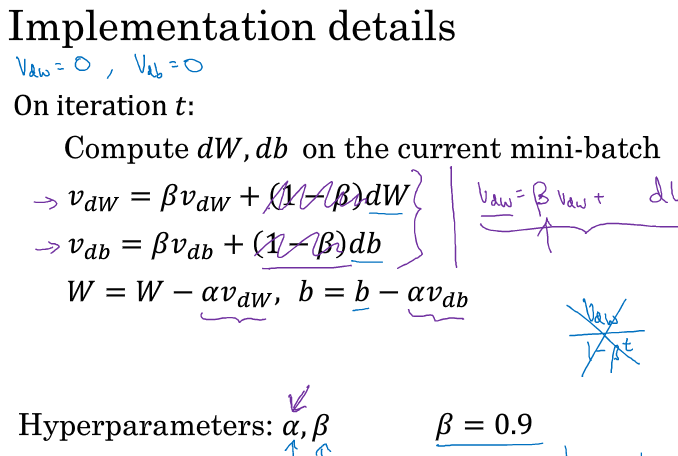指数加权平均  Exponentially weighted averages

这里补充一下指数加权平均的概念。课堂上是以伦敦连续很多天的温度为例子，是指第t天的温度

这里的指数加权平均Vt大约是  天的平均温度

### 2、自适应学习率算法

• RMSprop

和Momentum算法一样，可以消除梯度下降中的摆动并使用更大的学习率。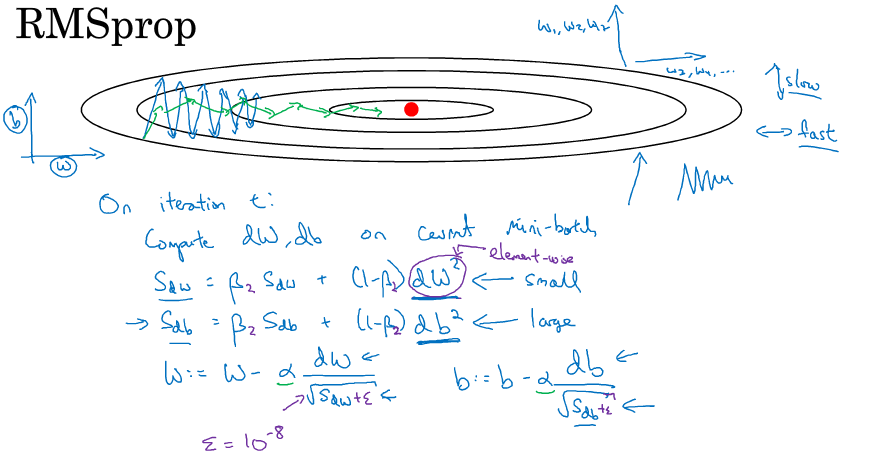结合了Momentum和RMSprop两种算法，是很常用的优化算法。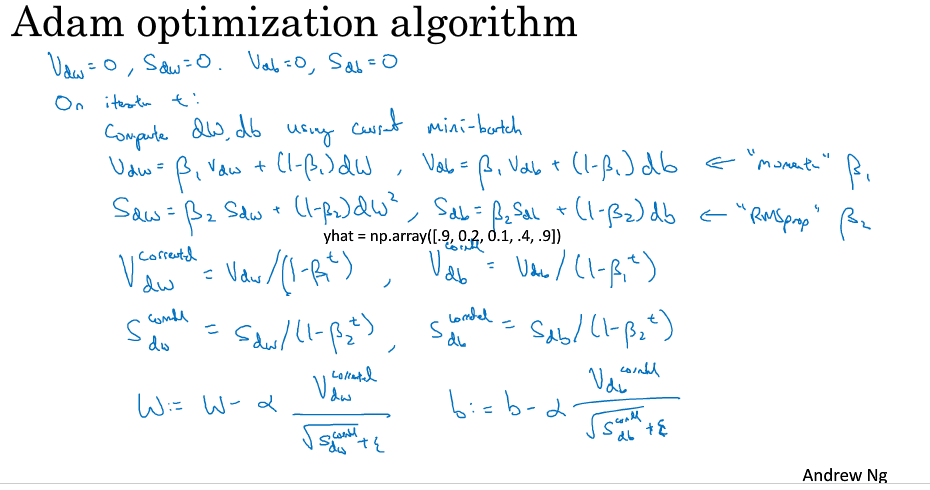# 四、优化策略

许多优化技术并非真正的算法，而是一般化的模板，可以特定地产生算法，或是并入到很多不同的算法中。

• 正则化输入 Normalizing inputs

正则化输入可以使得代价函数更圆，从而加快训练速度。

实现方法分为两步：零均值化，归一化方差。即减去均值除以标准差。

• 批标准化   Batch Normalization

​​​​​​​       批标准化使得每一层的隐藏单元有着标准的均值和方差（不一定分别为0和1），从而加快训练速度。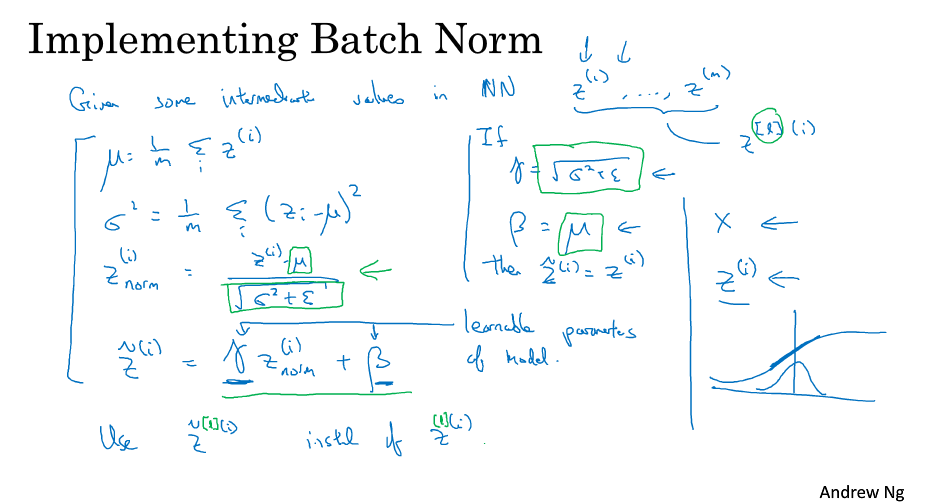这两个参数是需要学习的参数。更多内容参看论文。

• 预训练

待学习

• 学习率衰减 Learning rate decay

​​​​​​​

两个超参数：decayrate、

【2】《深度学习总结(五)——各优化算法》https://blog.csdn.net/manong_wxd/article/details/78735439

【3】《深度学习常用优化算法》https://blog.csdn.net/pandamax/article/details/72852960

2018-05-02 06:32:19 qq_39422642 阅读数 1853
• ###### 基于深度学习实现场景判断模型和算法优化

本次课程诚邀PowerAI人工智能马拉松编程大赛获奖前三名团队精英，在线分享大赛获奖作品算法/模型等技术干货。

3682 人正在学习 去看看 CSDN讲师

## 4.1 动量momentum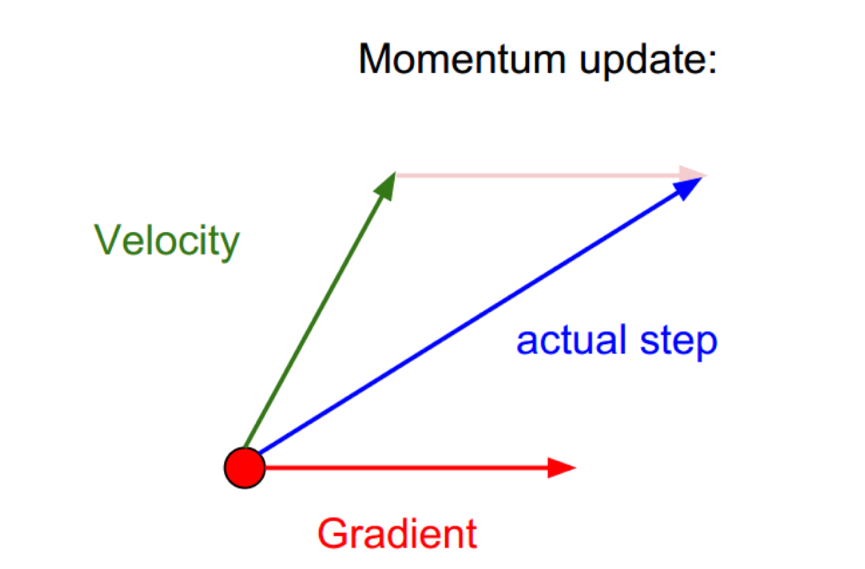$g={\mathrm{\nabla }}_{w}\left(\frac{1}{m}\sum _{i}^{m}L\left(f\left({x}^{\left(i\right)};w\right),{y}^{\left(i\right)}\right)\right)$

$v=\alpha v-\epsilon g$

$w=w+v$

$v=\alpha v-\epsilon {\mathrm{\nabla }}_{w}\left(\frac{1}{m}\sum _{i}^{m}L\left(f\left({x}^{\left(i\right)};w+\alpha v\right),{y}^{\left(i\right)}\right)\right)$

$r=r+g\odot g$
,然后得到参数更新的公式：
$w=w-\frac{\epsilon }{\delta +\sqrt{r}}\odot g$

## 4.3 RMSProp

Hinton提出的RMSProp是Adadelta的一个特例，我们通过它了解一下具体是怎么计算的。RMSProp旨在使用指数衰减平均来丢弃非常遥远的历史梯度,相当于指数加权平均来计算梯度。假设衰减速率为$\rho$$\rho$,那么累积平方梯度可以表示为：

$r=\rho r+\left(1-\rho \right)g\odot g$

$w=w-\frac{\epsilon }{\sqrt{\delta +r}}\odot g$

$s={\rho }_{1}s+\left(1-{\rho }_{1}\right)g$

$r={\rho }_{2}r+\left(1-{\rho }_{2}\right)g\odot g$

$\stackrel{^}{s}=\frac{s}{1-{\rho }_{1}^{t}},\stackrel{^}{r}=\frac{r}{1-{\rho }_{2}^{t}}$

$w=w-\epsilon \frac{\stackrel{^}{s}}{\sqrt{\stackrel{^}{r}}+\delta }$

## 4.5 几种算法的比较

s = rho1*s + (1-rho1)*g
st = s/(1-rho1**t)

r = rho2*r + (1 - rho2)*(g**2)
rt = r/(1-rho2**t)

w += - learning_rate*st/(np.sqrt(rt) + delta)

r = r + g**2
w += -learning_rate*g/(np.sqrt(r) + delta)

r = decay_rate*r + (1-decay_rate)*g**2
w += -learning_rate*g/(np.sqrt(r + delta))可以发现，梯度下降法在鞍点上徘徊了很长一段时间，才找到正确的迭代方向，而RMSProp则很快就找到了下降的方向了，进一步说明该算法的实用性。

Unit Tests for Stochastic Optimization
cs231n
deeplearning

2019-04-09 14:08:08 weixin_41665360 阅读数 358
• ###### 基于深度学习实现场景判断模型和算法优化

本次课程诚邀PowerAI人工智能马拉松编程大赛获奖前三名团队精英，在线分享大赛获奖作品算法/模型等技术干货。

3682 人正在学习 去看看 CSDN讲师

## 1、梯度下降

### 1.1、Batch 梯度下降

$Batch$ 梯度下降计算整个训练集的成本函数相对参数 $θ$ 的梯度
$\theta^{t+1}=\theta^t-\eta \cdot \nabla_{\theta} J(\theta^t)\tag1$

### 1.2、随机梯度下降（SGD）

$\theta^{t+1}=\theta^t-\eta \cdot \nabla_{\theta} J\left(\theta^t ; x_i ; y_i\right)\tag2$
$Batch$ 梯度下降对大数据集执行冗余计算，因为它在每个参数更新前重新计算相似样本梯度。$SGD$ 一次只执行一个样本上的更新来消除这种冗余。因此它通常更快，而且可以用于在线学习$SGD$ 以很大的方差执行频繁的更新，导致目标函数剧烈波动，如图 $1$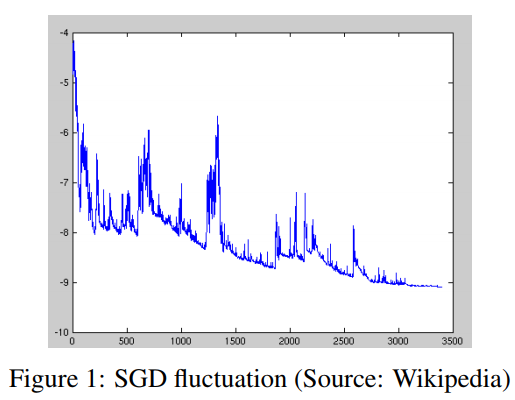$Batch$ 梯度下降能够将参数更新到极小值。$SGD$ 的波动性一方面使它能够跳转到新的、可能更好的局部极小值，另一方面，这使收敛到精确的最小值变得复杂，因为 $SGD$ 会在最小值附近振荡。但是，当缓慢减小学习率时，$SGD$ 表现出与 $Batch$ 梯度下降相同的收敛性，几乎确定收敛到非凸优化的局部最小值和凸优化的全局最小值。

### 1.3、Mini-batch 梯度下降

$Mini-batch$ 梯度下降对两种方法进行折中，实现了最佳效果，并为每一个 $mini-batch$$n$ 个样本执行一次更新。
$\theta^{t+1}=\theta^t-\eta \cdot \nabla_{\theta} J\left(\theta^t ; x_{(i : i+n)} ; y_{(i : i+n)}\right)\tag3$

1. 减小参数更新的方差，使收敛过程更稳定
2. 利用常见的深度学习库的高度优化的矩阵计算方法，高效计算 $mini-batch$ 梯度

1. 选择合适的学习率很困难。学习率太小会导致收敛速度过慢，学习率太大会阻碍收敛，导致损失函数在最小值附近波动，甚至发散
2. 学习率 $schedules$ 尝试调整训练时的学习率，比如退火，即根据预定义的 $schedules$ 或者当迭代之间的目标变化低于某一阈值时，减小学习率。但是，$schedules$ 或者阈值必须预定义，因此无法适应数据集特征
3. 所有参数更新使用相同学习率。如果数据是稀疏的，而且特征频率差异较大，那么我们不想同等程度更新所有参数，而是对较少出现的特征执行更大的更新
4. 最小化高度非凸误差函数的另一个关键挑战是避免陷入许多次优局部极小$Dauphin$ 等人认为，造成这一困难的原因不是局部极小值，而是鞍点，即在一个维度上坡度增加，另一维度上坡度减小。$SGD$ 在这些鞍点处很难逃脱，因为在所有维度上的梯度都接近于 $0$

## 2、梯度下降优化算法

### 2.1、Momentum

$SGD$ 很难摆脱沟壑，即曲面的曲线在一个维度比另一维度更平稳，这通常是在局部最优附近。在这些场景下，$SGD$ 在峡谷的斜坡上振荡，沿着底部向局部最优方向前进的过程中徘徊不前，如图 $2a$ 所示。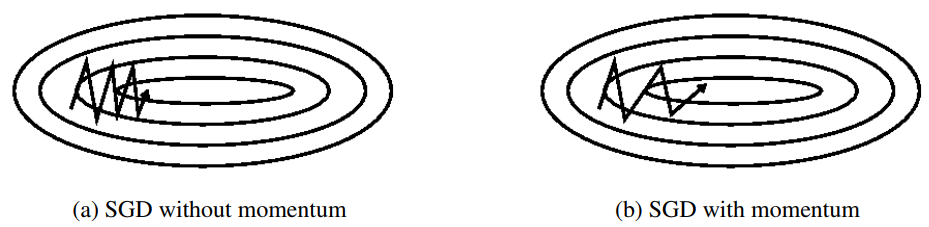$Momentum$ 有助于加速相关方向的梯度下降，并抑制振荡，如图 $2b$ 所示。它通过将之前的更新向量的 $\gamma$ 倍加到当前更新向量来实现这一点。
\begin{aligned} v^{t} &=\gamma v^{t-1}+\eta \nabla_{\theta} J(\theta^t) \\ \theta^{t+1} &=\theta^t-v^t \end{aligned}\tag4

### 2.2、Nesterov 加速梯度

$NAG$ 是一种给出预见性动量项的方法。由于已知使用动量项 $\gamma v^{t-1}$ 来更新参数，因此计算 $\theta^t-\gamma v^{t-1}$ 给出了参数下一个位置的近似。这样做的好处是算法可以提前知道梯度方向发生改变，并相应地减小更新步长，从而加速收敛
\begin{aligned} v^{t} &=\gamma v^{t-1}+\eta \nabla_{\theta} J\left(\theta^t-\gamma v^{t-1}\right) \\ \theta^{t+1} &=\theta^t-v^{t} \end{aligned}\tag5
$\gamma$ 设为 $0.9$$Momentum$ 首先计算当前梯度（图 $3$ 小蓝色向量）然后在更新的累积梯度方向上前进一大步（大蓝色向量），$NAG$ 首先在之前累积的梯度方向进行大的跳跃（棕色向量），测量梯度然后进行校正（绿色向量）。这一预见性更新防止了参数更新过快并提升了响应能力，极大提升了 $RNN$ 在许多任务上的性能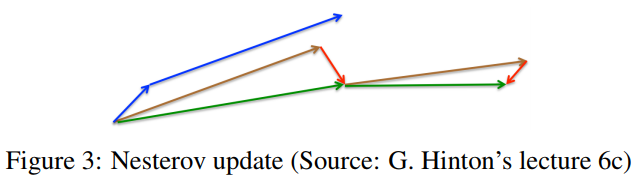$Keras$ 中调用相关优化器方法

keras.optimizers.SGD(lr=0.01, momentum=0.0, decay=0.0, nesterov=False)
"""
: lr: 学习率 >= 0的浮点数
: momentum: >= 0的浮点数，在相关方向加速 SGD 并抑制震荡
: decay:  >= 0的浮点数，学习率随着更新过程衰减
: nesterov: 布尔值，是否应用 Nesterov 动量，一般用于 RNN
"""


$Adagrad$ 是一种基于梯度的优化算法，它使学习率适应于参数，对低频参数执行更大的更新，对高频参数执行更小的更新。因此，它非常适合处理稀疏数据$Adagrad$ 极大地提升了 $SGD$ 的鲁棒性。

$g_i^t=\nabla_{\theta^t} J\left(\theta_i^t\right)\tag6$
$\theta_i^{t+1}=\theta_i^{t}-\frac{\eta}{\sqrt{G_{i i}^t+\epsilon}} \cdot g_i^t\tag7$

$\theta^{(t+1)}=\theta^{(t)}-\eta\left(G^{t}\right)^{-1}\odot\nabla_{\theta^t} J\left(\theta^t\right)\tag8$

$G^t=\left[\begin{matrix}\sqrt{\sum\limits_{\tau=1}^tg_1^{\tau}+\epsilon}&0&\cdots&0\\0&\sqrt{\sum\limits_{\tau=1}^tg_2^{\tau}+\epsilon}&\cdots&0\\\vdots&\vdots&\ddots&\vdots\\0&0&\cdots&\sqrt{\sum\limits_{\tau=1}^tg_d^{\tau}+\epsilon}\end{matrix}\right]$
$G^t$ 是一个对角矩阵，每一个对角线元素为该位置对应参数的前 $t$ 次更新中梯度的平方和$\epsilon$ 为平滑参数，避免分母为 $0$（通常为 $1e-8$）。有趣的是，如果没有平方根运算，该算法效果更差

$Adagrad$ 主要的优点是它不需要手动调整学习率，通常使用默认值 $0.01$

$Adagrad$ 主要的缺点是，它在分母中积累了平方梯度：由于增加的每一项都是正的，训练过程中累计和不断增加。这相应地导致学习率减小，最终趋于无穷小，此时算法难以继续学习

$Keras$ 调用优化器方法：

keras.optimizers.Adagrad(lr=0.01, epsilon=1e-06)
"""
lr：大于0的浮点数，学习率
epsilon：大于0的小浮点数，防止除0错误
"""


$Adadelta$$Adagrad$ 的延伸。取代累加之前所有梯度平方，$Adadelta$ 累加之前固定范围 $w$ 内的梯度。

$E\left[g^{2}\right]_{t}=\gamma E\left[g^{2}\right]_{t-1}+(1-\gamma) g_{t}^{2}\tag{10}$
$\gamma$ 设为与动量项类似的值，约为 $0.9$。为清晰起见，根据参数更新向量 $\Delta\theta_t$ 重写 $SGD$ 更新。
\begin{aligned} \Delta \theta_{t} &=-\eta \cdot g_{t, i} \\ \theta_{t+1} &=\theta_{t}+\Delta \theta_{t} \end{aligned}\tag{11}
$Adagrad$ 的参数更新向量表示为：
$\Delta \theta_{t}=-\frac{\eta}{\sqrt{G_{t}+\epsilon}} \odot g_{t}\tag{12}$

$\Delta \theta_{t}=-\frac{\eta}{\sqrt{E\left[g^{2}\right]_{t}+\epsilon}} g_{t}\tag{13}$

$\Delta \theta_{t}=-\frac{\eta}{R M S[g]_{t}} g_{t}\tag{14}$

$E\left[\Delta \theta^{2}\right]_{t}=\gamma E\left[\Delta \theta^{2}\right]_{t-1}+(1-\gamma) \Delta \theta_{t}^{2}\tag{15}$

$RMS[\Delta \theta]_{t}=\sqrt{E\left[\Delta \theta^{2}\right]_{t}+\epsilon}\tag{16}$

\begin{aligned} \Delta \theta_{t} &=-\frac{R M S[\Delta \theta]_{t-1}}{R M S[g]_{t}} g_{t} \\ \theta_{t+1} &=\theta_{t}+\Delta \theta_{t} \end{aligned}\tag{17}

$Keras$ 调用优化器方法：

keras.optimizers.Adadelta(lr=1.0, rho=0.95, epsilon=1e-06)
"""
lr：大于0的浮点数，学习率
rho：大于0的浮点数
epsilon：大于0的小浮点数，防止除0错误
"""


### 2.5、RMSprop

$RMSprop$ 是一种自适应学习率方法。它与 $Adadelta$ 均用于解决 $Adagrad$ 学习率急剧下降的问题。事实上，$RMSprop$$Adadelta$ 的第一个更新向量相同。
\begin{aligned} E\left[g^{2}\right]_{t} &=0.9 E\left[g^{2}\right]_{t-1}+0.1 g_{t}^{2} \\ \theta_{t+1} &=\theta_{t}-\frac{\eta}{\sqrt{E\left[g^{2}\right]_{t}+\epsilon}} g_{t} \end{aligned}\tag{18}

$Keras$ 调用优化器方法：

keras.optimizers.RMSprop(lr=0.001, rho=0.9, epsilon=1e-06)
"""
lr：大于0的浮点数，学习率
rho：大于0的浮点数
epsilon：大于0的小浮点数，防止除0错误
"""


$Adaptive~Moment~Estimation(Adam)$ 是另一种计算每个参数自适应学习率的方法。除了存储指数衰减的平方梯度均值 $v_t$，它还保留了类似于 $momentum$ 的，指数衰减的梯度均值 $m_t$
\begin{aligned} m_{t} &=\beta_{1} m_{t-1}+\left(1-\beta_{1}\right) g_{t} \\ v_{t} &=\beta_{2} v_{t-1}+\left(1-\beta_{2}\right) g_{t}^{2} \end{aligned}\tag{19}
$m_t$$v_t$ 分别为梯度的一阶矩（均值）和二阶矩估计，这是该方法名字的由来。当 $m_t$$v_t$ 被初始化为零向量时，在初始时间步，它们都偏向于 $0$，特别当衰变率很小时更是如此（即，$\beta_1$$\beta_2$ 接近于 $1$）。

\begin{aligned} \hat{m}_{t} &=\frac{m_{t}}{1-\beta_{1}^{t}} \\ \hat{v}_{t} &=\frac{v_{t}}{1-\beta_{2}^{t}} \end{aligned}\tag{20}
$Adam$ 更新规则如下：
$\theta_{t+1}=\theta_{t}-\frac{\eta}{\sqrt{\hat{v}_{t}}+\epsilon} \hat{m}_{t}\tag{21}$
$\beta_1$ 默认为 $0.9$$\beta_2$ 默认为 $0.999$$\epsilon$ 默认为 $10^{-8}$。实验表明，相比于其他自适应算法，$Adam$ 效果更好。

$Keras$ 调用优化器方法：

keras.optimizers.Adam(lr=0.001, beta_1=0.9, beta_2=0.999, epsilon=1e-08)
"""
lr：大于0的浮点数，学习率
beta_1/beta_2：浮点数， 0<beta<1，通常很接近1
epsilon：大于0的小浮点数，防止除0错误
"""


$Adam$ 中的 $v_t$ 因子反向缩放之前梯度的 $\ell_2$ 范数和当前梯度 $|g_t|^2$
$v_{t}=\beta_{2} v_{t-1}+\left(1-\beta_{2}\right)\left|g_{t}\right|^{2}\tag{22}$

$v_{t}=\beta_{2}^{p} v_{t-1}+\left(1-\beta_{2}^{p}\right)\left|g_{t}\right|^{p}\tag{23}$
$p$ 值范数通常在数值上不稳定，因此实践中 $\ell_1$$\ell_2$ 范数比较常见。然而，$\ell_{\infty}$ 通常表现更稳定。因此作者提出了 $AdaMax$，并证明了具有 $\ell_{\infty}$$v_t$ 收敛到更稳定的值。为避免与 $adam$ 混淆，这里使用 $u_t$ 表示受无穷范数约束的 $v_t$
\begin{aligned} u_{t} &=\beta_{2}^{\infty} v_{t-1}+\left(1-\beta_{2}^{\infty}\right)\left|g_{t}\right|^{\infty} \\ &=\max \left(\beta_{2} \cdot v_{t-1},\left|g_{t}\right|\right) \end{aligned}\tag{24}

$\theta_{t+1}=\theta_{t}-\frac{\eta}{u_{t}} \hat{m}_{t}\tag{25}$

$Keras$ 调用优化器方法：

keras.optimizers.Adamax(lr=0.002, beta_1=0.9, beta_2=0.999, epsilon=1e-08)
"""
lr：大于0的浮点数，学习率
beta_1/beta_2：浮点数， 0<beta<1，通常很接近1
epsilon：大于0的小浮点数，防止除0错误
"""


$Adam$ 可以看作 $RMSprop$$momentum$ 的结合$RMSprop$ 贡献指数衰减的之前梯度平方的均值 $v_t$$momentum$ 计算指数衰减的之前梯度的均值 $m_t$$Nesterov$ 加速梯度优于普通的 $momentum$

$Nadam(Nesterov-accelerated~Adaptive~Moment~Estimation)$$Adam$$NAG$ 结合起来。为了将 $NAG$ 结合到 $Adam$ 需要修改动量项 $m_t$

\begin{aligned} g_{t} &=\nabla_{\theta_{t}} J\left(\theta_{t}\right) \\ m_{t} &=\gamma m_{t-1}+\eta g_{t} \\ \theta_{t+1} &=\theta_{t}-m_{t} \end{aligned}\tag{26}

$\theta_{t+1}=\theta_{t}-\left(\gamma m_{t-1}+\eta g_{t}\right)\tag{27}$
$NAG$ 在计算梯度之前，使用动量步长更新参数，从而实现更精确的参数更新。
\begin{aligned} g_{t} &=\nabla_{\theta_{t}} J\left(\theta_{t}-\gamma m_{t-1}\right) \\ m_{t} &=\gamma m_{t-1}+\eta g_{t} \\ \theta_{t+1} &=\theta_{t}-m_{t} \end{aligned}\tag{28}
$Dozat$ 提出修改 $NAG$：不再使用两次动量—一次用于更新梯度，另一次用于更新参数。直接使用前瞻性动量向量更新当前参数。
\begin{aligned} g_{t} &=\nabla_{\theta_{t}} J\left(\theta_{t}\right) \\ m_{t} &=\gamma m_{t-1}+\eta g_{t} \\ \theta_{t+1} &=\theta_{t}-\left(\gamma m_{t}+\eta g_{t}\right) \end{aligned}\tag{29}

\begin{aligned} m_{t} &=\beta_{1} m_{t-1}+\left(1-\beta_{1}\right) g_{t} \\ \hat{m}_{t} &=\frac{m_{t}}{1-\beta_{1}^{t}} \\ \theta_{t+1} &=\theta_{t}-\frac{\eta}{\sqrt{\hat{v}_{t}}+\epsilon} \hat{m}_{t} \end{aligned}\tag{30}

$\theta_{t+1}=\theta_{t}-\frac{\eta}{\sqrt{\hat{v}_{t}}+\epsilon}\left(\frac{\beta_{1} m_{t-1}}{1-\beta_{1}^{t}}+\frac{\left(1-\beta_{1}\right) g_{t}}{1-\beta_{1}^{t}}\right)\tag{31}$

$\theta_{t+1}=\theta_{t}-\frac{\eta}{\sqrt{\hat{v}_{t}}+\epsilon}\left(\beta_{1} \hat{m}_{t-1}+\frac{\left(1-\beta_{1}\right) g_{t}}{1-\beta_{1}^{t}}\right)\tag{32}$

$\theta_{t+1}=\theta_{t}-\frac{\eta}{\sqrt{\hat{v}_{t}}+\epsilon}\left(\beta_{1} \hat{m}_{t}+\frac{\left(1-\beta_{1}\right) g_{t}}{1-\beta_{1}^{t}}\right)\tag{33}$

$Keras$ 调用优化器方法：

keras.optimizers.Nadam(lr=0.002, beta_1=0.9, beta_2=0.999,
epsilon=1e-08, schedule_decay=0.004)
"""
lr：大于0的浮点数，学习率
beta_1/beta_2：浮点数， 0<beta<1，通常很接近1
epsilon：大于0的小浮点数，防止除0错误
"""



### 2.9、各优化方法收敛速度的比较### 2.10、如何选择优化方法

1. 如果数据是稀疏的，就选择自适应学习率方法。还可以省去调节学习率的麻烦
2. $RMSprop$$Adagrad$ 的扩展，解决学习率急剧消失的问题
3. $Adadelta$$RMSprop$ 相同，只不过在更新规则的分子上使用了参数更新均方根
4. $Adam$$RMSprop$ 的基础上使用了偏移校正和动量
5. $RMSprop$$Adadelta$$Adam$ 是相似的算法，在类似的情况的下做的很好。优化快要结束时，梯度变得越来越稀疏，偏移校正使 $Adam$ 略微超过 $RMSprop$目前来说，$Adam$ 可能是整体优化的最佳选择
6. 训练较深较复杂的网络时，推荐使用学习率自适应的优化方法。

## 3、参考

An overview of gradient descent optimization
algorithms